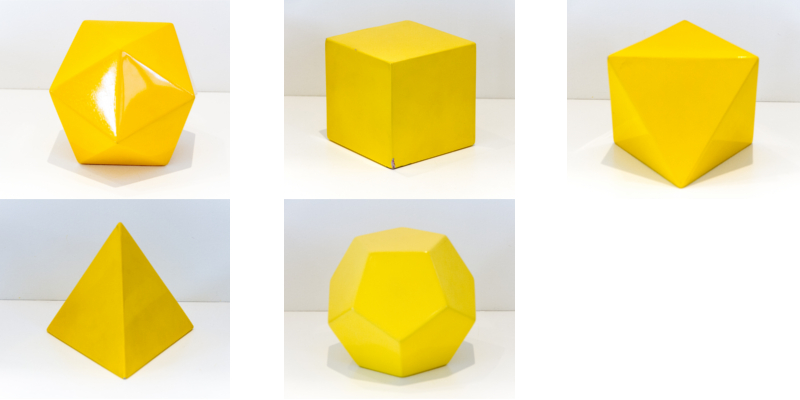## POLYHEDRAKepler studied polyhedra a lot. His fascination was so great that he tried to explain the distances of the planets from the Sun on the basis of a scheme of spheres and polyhedra inscribed on each other, in his book Mysterium Cosmographicum.

Interest in polyhedrons dates back to Ancient Greece and continues to this day. To date, there is research being done on polyhedra and also polytopes, which are their generalization to higher dimensions and to non-Euclidean geometries, such as hyperbolic or spherical.

In addition to aesthetic pleasure, polyhedra provided beautiful, simple and surprising theorems. After the Renaissance, we highlight Euler's Theorem, relating the number of faces, edges and vertices of a polyhedron by the formula V+F=A+2.

Euler's formula is valid as long as the polyhedron is equivalent, in the topological sense, to a sphere. It means, intuitively, that we can “inflate” the polyhedron until it becomes a sphere.

For polyhedra of this type, Descartes' Formula is also valid, which states that the sum of the angular deficiencies of the vertices is always equal to 4π (or 720 degrees). The angular deficiency of a vertex is how far it takes for the sum of face angles incident on that vertex to reach 2π (or 360 degrees).

## CONVEX POLYHEDRA WITH REGULAR FACES

UNIFORM POLYHEDRA Uniform polyhedra are those in which all vertices are indistinguishable from each other. One can say that 'what you see of the polyhedron from a vertex is exactly what you see from any other vertex'. They are classified into 'platonic', 'prisms', 'antiprisms', and 'archimedean'.

PLATONIC These are the uniform polyhedra with equal faces (tetrahedron, cube, octahedron, dodecahedron and icosahedron).PRISMS Parallel bases connected by squares (infinite family).

ARCHIMEDEANS All other uniform polyhedra (finitely many).

NON-UNIFORMS Pyramids, domes, deltahedrons, cuts of uniforms etc. They can be both elementary and composite - composite polyhedra are those which may be split by a plane into two other convex polyhedra with regular faces, while the elementary ones can't. They are finitely many.

If you want to know more about these amazing subject, watch the two videos above! In the video "Plato's Polyhedrons: Dual and Inscribed, Profesor Doctor Lucia Satie Ikemoto Murakami mentions some plans, which are available on the Symmetries page. Besides, seize the opportunity to check the other cool videos in the Matemateca's channel on YouTube!